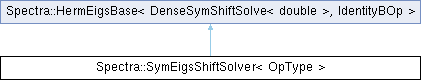Spectra  1.0.1 Header-only C++ Library for Large Scale Eigenvalue Problems
Spectra::SymEigsShiftSolver< OpType > Class Template Reference

#include <Spectra/SymEigsShiftSolver.h>

Inheritance diagram for Spectra::SymEigsShiftSolver< OpType >:## Public Member Functions

SymEigsShiftSolver (OpType &op, Index nev, Index ncv, const Scalar &sigma)Public Member Functions inherited from Spectra::SymEigsBase< DenseSymShiftSolve< double >, IdentityBOp >
void init (const Scalar *init_resid)

void init ()

Index compute (SortRule selection=SortRule::LargestMagn, Index maxit=1000, Scalar tol=1e-10, SortRule sorting=SortRule::LargestAlge)

CompInfo info () const

Index num_iterations () const

Index num_operations () const

Vector eigenvalues () const

virtual Matrix eigenvectors (Index nvec) const

virtual Matrix eigenvectors () const

## Detailed Description

### template<typename OpType = DenseSymShiftSolve<double>> class Spectra::SymEigsShiftSolver< OpType >

This class implements the eigen solver for real symmetric matrices using the shift-and-invert mode. The background information of the symmetric eigen solver is documented in the SymEigsSolver class. Here we focus on explaining the shift-and-invert mode.

The shift-and-invert mode is based on the following fact: If $$\lambda$$ and $$x$$ are a pair of eigenvalue and eigenvector of matrix $$A$$, such that $$Ax=\lambda x$$, then for any $$\sigma$$, we have

$(A-\sigma I)^{-1}x=\nu x$

where

$\nu=\frac{1}{\lambda-\sigma}$

which indicates that $$(\nu, x)$$ is an eigenpair of the matrix $$(A-\sigma I)^{-1}$$.

Therefore, if we pass the matrix operation $$(A-\sigma I)^{-1}y$$ (rather than $$Ay$$) to the eigen solver, then we would get the desired values of $$\nu$$, and $$\lambda$$ can also be easily obtained by noting that $$\lambda=\sigma+\nu^{-1}$$.

The reason why we need this type of manipulation is that the algorithm of Spectra (and also ARPACK) is good at finding eigenvalues with large magnitude, but may fail in looking for eigenvalues that are close to zero. However, if we really need them, we can set $$\sigma=0$$, find the largest eigenvalues of $$A^{-1}$$, and then transform back to $$\lambda$$, since in this case largest values of $$\nu$$ implies smallest values of $$\lambda$$.

To summarize, in the shift-and-invert mode, the selection rule will apply to $$\nu=1/(\lambda-\sigma)$$ rather than $$\lambda$$. So a selection rule of LARGEST_MAGN combined with shift $$\sigma$$ will find eigenvalues of $$A$$ that are closest to $$\sigma$$. But note that the eigenvalues() method will always return the eigenvalues in the original problem (i.e., returning $$\lambda$$ rather than $$\nu$$), and eigenvectors are the same for both the original problem and the shifted-and-inverted problem.

Template Parameters
 OpType The name of the matrix operation class. Users could either use the wrapper classes such as DenseSymShiftSolve and SparseSymShiftSolve, or define their own that implements the type definition Scalar and all the public member functions as in DenseSymShiftSolve.

Below is an example that illustrates the use of the shift-and-invert mode:

#include <Eigen/Core>
#include <Spectra/SymEigsShiftSolver.h>
// <Spectra/MatOp/DenseSymShiftSolve.h> is implicitly included
#include <iostream>
using namespace Spectra;
int main()
{
// A size-10 diagonal matrix with elements 1, 2, ..., 10
Eigen::MatrixXd M = Eigen::MatrixXd::Zero(10, 10);
for (int i = 0; i < M.rows(); i++)
M(i, i) = i + 1;
// Construct matrix operation object using the wrapper class
DenseSymShiftSolve<double> op(M);
// Construct eigen solver object with shift 0
// This will find eigenvalues that are closest to 0
SymEigsShiftSolver<DenseSymShiftSolve<double>> eigs(op, 3, 6, 0.0);
eigs.init();
eigs.compute(SortRule::LargestMagn);
if (eigs.info() == CompInfo::Successful)
{
Eigen::VectorXd evalues = eigs.eigenvalues();
// Will get (3.0, 2.0, 1.0)
std::cout << "Eigenvalues found:\n" << evalues << std::endl;
}
return 0;
}
@ Successful
Computation was successful.

Also an example for user-supplied matrix shift-solve operation class:

#include <Eigen/Core>
#include <Spectra/SymEigsShiftSolver.h>
#include <iostream>
using namespace Spectra;
// M = diag(1, 2, ..., 10)
class MyDiagonalTenShiftSolve
{
private:
double sigma_;
public:
using Scalar = double; // A typedef named "Scalar" is required
int rows() const { return 10; }
int cols() const { return 10; }
void set_shift(double sigma) { sigma_ = sigma; }
// y_out = inv(A - sigma * I) * x_in
// inv(A - sigma * I) = diag(1/(1-sigma), 1/(2-sigma), ...)
void perform_op(double *x_in, double *y_out) const
{
for (int i = 0; i < rows(); i++)
{
y_out[i] = x_in[i] / (i + 1 - sigma_);
}
}
};
int main()
{
MyDiagonalTenShiftSolve op;
// Find three eigenvalues that are closest to 3.14
SymEigsShiftSolver<MyDiagonalTenShiftSolve> eigs(op, 3, 6, 3.14);
eigs.init();
eigs.compute(SortRule::LargestMagn);
if (eigs.info() == CompInfo::Successful)
{
Eigen::VectorXd evalues = eigs.eigenvalues();
// Will get (4.0, 3.0, 2.0)
std::cout << "Eigenvalues found:\n" << evalues << std::endl;
}
return 0;
}

Definition at line 149 of file SymEigsShiftSolver.h.

## ◆ SymEigsShiftSolver()

template<typename OpType = DenseSymShiftSolve<double>>
 Spectra::SymEigsShiftSolver< OpType >::SymEigsShiftSolver ( OpType & op, Index nev, Index ncv, const Scalar & sigma )
inline

Constructor to create a eigen solver object using the shift-and-invert mode.

Parameters
 op The matrix operation object that implements the shift-solve operation of $$A$$: calculating $$(A-\sigma I)^{-1}v$$ for any vector $$v$$. Users could either create the object from the wrapper class such as DenseSymShiftSolve, or define their own that implements all the public members as in DenseSymShiftSolve. nev Number of eigenvalues requested. This should satisfy $$1\le nev \le n-1$$, where $$n$$ is the size of matrix. ncv Parameter that controls the convergence speed of the algorithm. Typically a larger ncv_ means faster convergence, but it may also result in greater memory use and more matrix operations in each iteration. This parameter must satisfy $$nev < ncv \le n$$, and is advised to take $$ncv \ge 2\cdot nev$$. sigma The value of the shift.

Definition at line 190 of file SymEigsShiftSolver.h.

The documentation for this class was generated from the following file: